Hyperhomology functor

(diff) ← Older revision | Latest revision (diff) | Newer revision → (diff)
A series of functors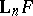on the category of complexes connected with some functor. In fact, let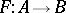be a covariant additive functor from an Abelian categorywith a sufficient number of projective objects into an Abelian category. Further, letbe a chain complex with values inand let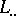be a Cartan–Eilenberg resolution of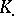, consisting of projective objects. Then the bicomplex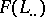determines the homology groups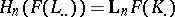and two spectral sequences (cf. Spectral sequence) converging to them with initial terms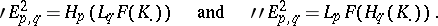These homology groups and spectral sequences depend functorially onand are known, respectively, as the hyperhomology functors forand the spectral hyperhomology functors for. The hyperhomology functor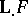is a homology functor on the category of complexes in the following important cases: whencommutes with inductive limits; when the objects in the categoryhave projective resolutions of length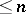; or when it is considered on the category of complexes with positive degrees.